# Vector

#### Resolution of Vectors

5. RESOLUTION OF VECTORS
(a) See the figure below. Vector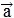is in x–y plane and it makes the angle α with the x–axis.(b)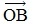andare the projection of vector on horizontal and vertical axis respectively.
(c) Applying trigonometric theory we can easily find out that OB = A cos α and BA = A sin α.
(d) So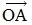=+=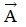= Ax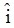+ Ay= A cos α+ A sin αIf you want to give information about online courses to other students, then share it with more and more on Facebook, Twitter, Google Plus. The more the shares will be, the more students will benefit. The share buttons are given below for your convenience.

#### IIT (Class X)

• Unit, Dimension & Error
• Vectors
• Motion in One Dimension
• PROJECTILE MOTION
• NEWTON'S LAWS OF MOTION & FRICTION
• WORK, POWER, ENERGY & CONSERVATION LAWS
• CIRCULAR MOTION & ROTATIONAL DYNAMICS
• GRAVITATION